## 基于照片对天线进行全波分析（详细分解步骤）

2023-07-31 10:37发布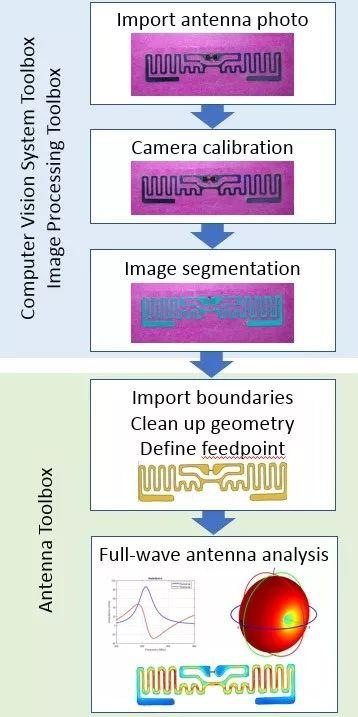RFID标签

c = webcam();

img = snapshot(c)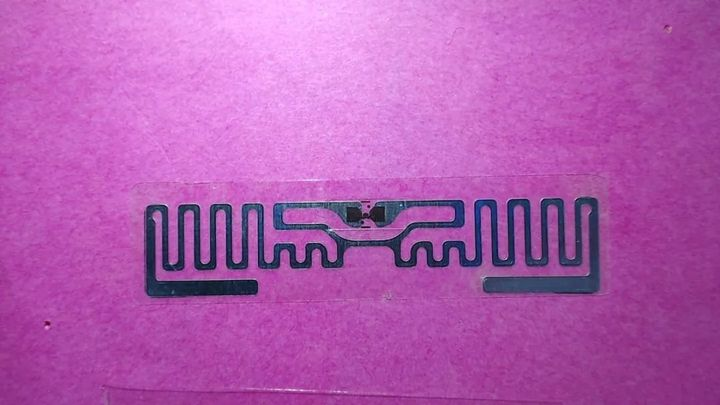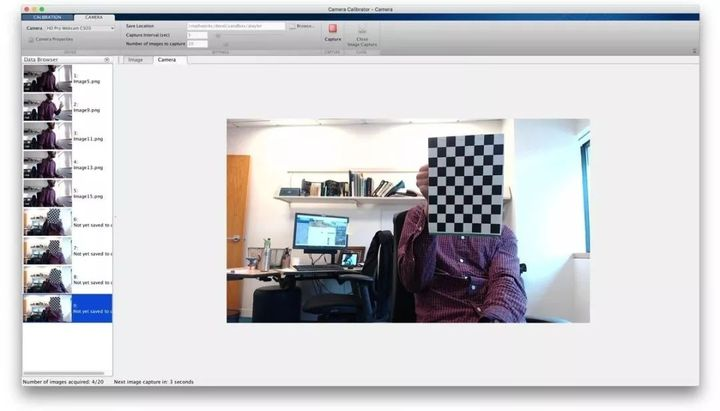undistortedImage = undistortImage(img,webcamParameters);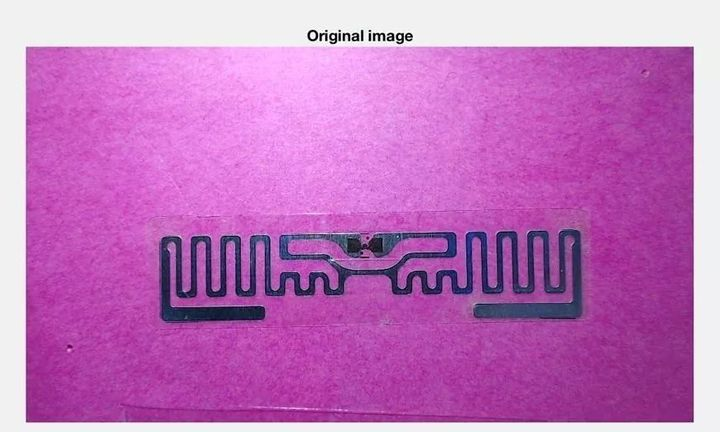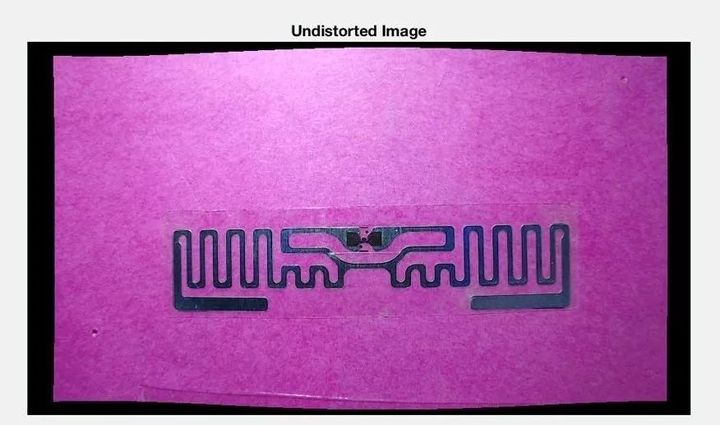Image Segmenter应用程序包含各种算法，它们可以按不同的方式组合使用，从而选择出分割对象的最佳方式。在本例中，我们将使用图形分割和一种称为“活动轮廓与蛇形轮廓”的迭代能量最小化算法。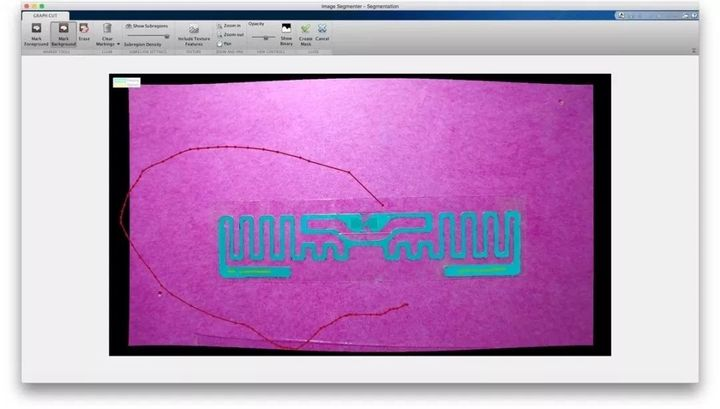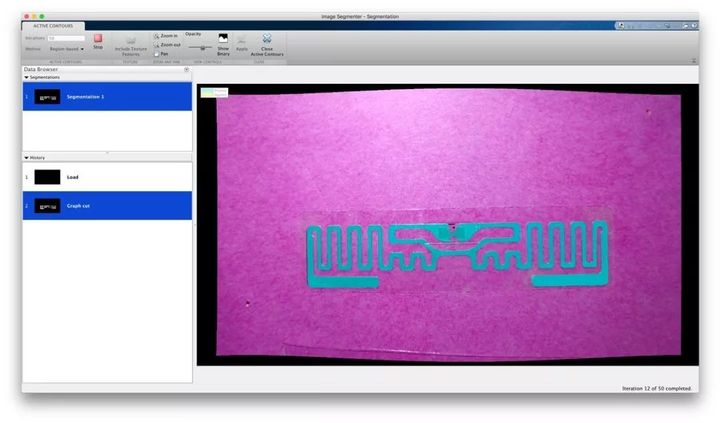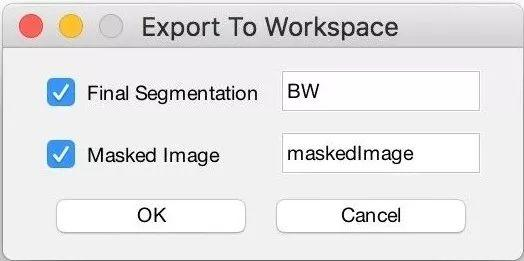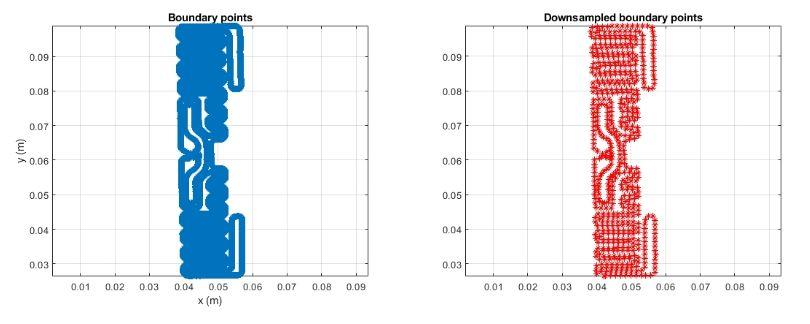outerPoly = antenna.Polygon;

outerPoly.Vertices = BpD{1};

innerPoly = antenna.Polygon;

innerPoly.Vertices = BpD{2};

c = outerPoly - innerPoly;

c = translate(c,[-(max(outerPoly.Vertices(:,1))-L/2),-(max(outerPoly.Vertices(:,2))-W/2),0]); figure

show(c)

title('RFID antenna geometry')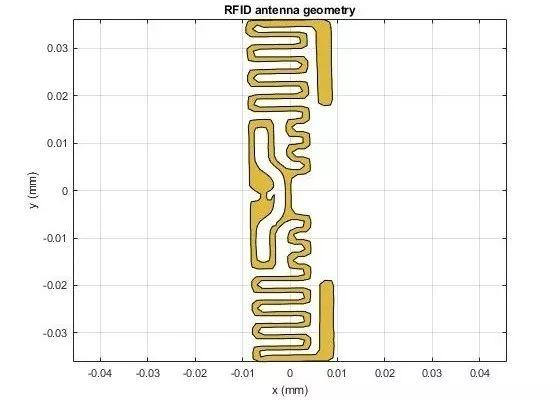gap = antenna.Rectangle('Length', 6e-3, 'Width', 2e-3, 'Center', [-5.5e-3 -1e-3]);

c = c - gap;

figure show(c) title('RFID antenna geometry with gap across feed region')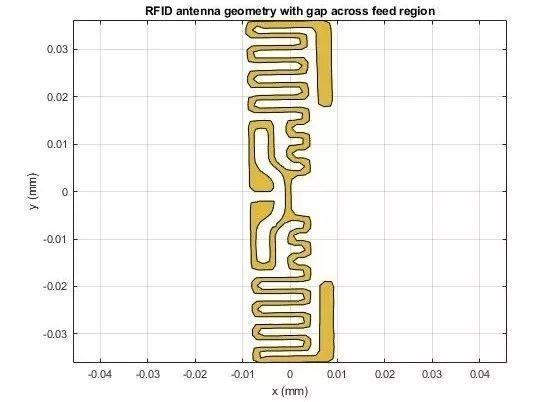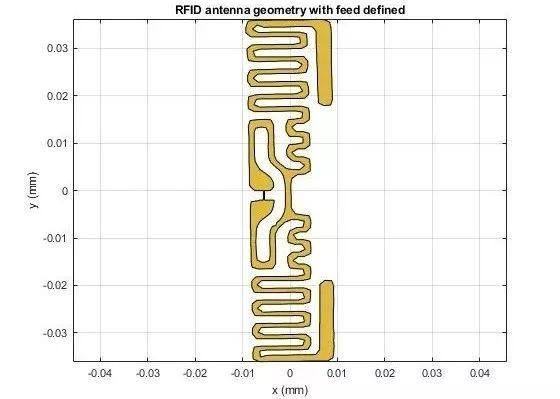feed = antenna.Rectangle('Length', 0.25e-3, 'Width', 3.0e-3, 'Center', [-5.5e-3, -1e-3]); cf = c + feed;

d = dielectric('Air');

p = pcbStack;

p.Name = 'RFID-tag';

p.BoardShape = antenna.Rectangle('Length',22e-3,'Width',80e-3);

p.Layers = {cf,d};

p.FeedLocations = [-5.5e-3, -1e-3, 1];

p.FeedDiameter = 0.5*0.25e-3;

figure

show(p)

view(0,90)

f_coarse = linspace(0.8e9,0.95e9,21);

figure

impedance(p, f_coarse)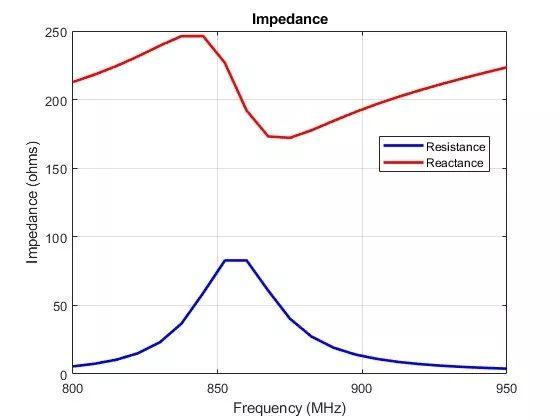figure

mesh(p)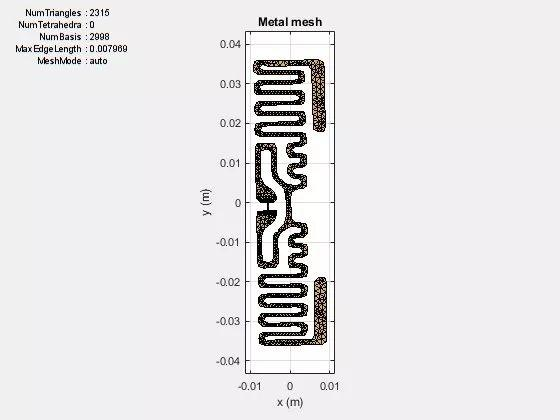X = -1i*200;

zl = lumpedElement;

zl.Impedance = X;

f_fine = linspace(0.8e9,0.95e9,51);

figure

impedance(p, f_fine)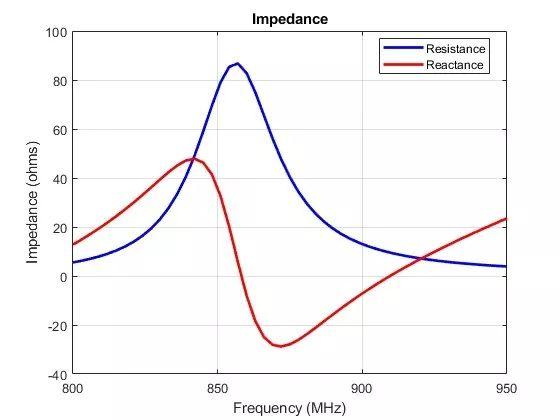figure

current(p,857e6)

view(0,90)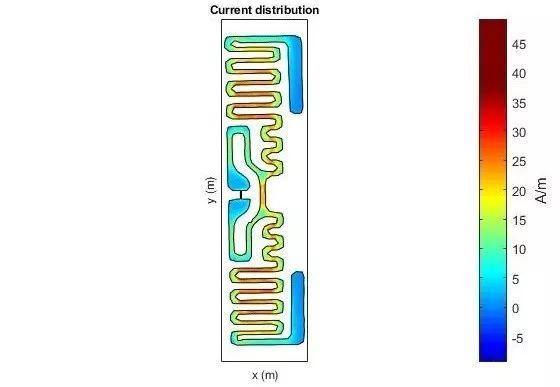RFID标签通常在一个平面中具有全向远场图案。为了证实这一点，我们将标签的远场辐射方向图进行可视化。该标签在857 MHz时的增益约为2 dBi。如图18所示，最大方向性出现在方位角为0度的仰角，沿着标签的最大尺寸的轴消失。天线的这种响应与在xy平面上与标签相同方向的简单半波长偶极子天线的响应类似。

figure

pattern(p,857e6)